Скачать презентацию Type I error control using law of iterated

f308ce5ba4ed5ec3c3be8fba832f838b.ppt

• Количество слайдов: 30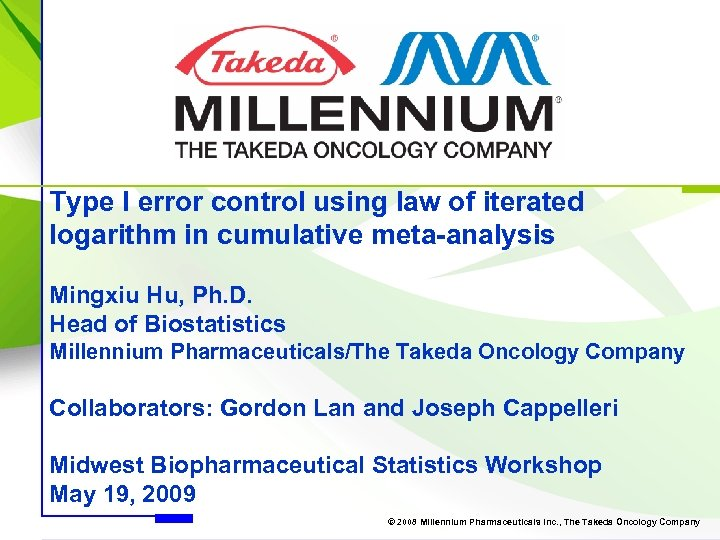Type I error control using law of iterated logarithm in cumulative meta-analysis Mingxiu Hu, Ph. D. Head of Biostatistics Millennium Pharmaceuticals/The Takeda Oncology Company Collaborators: Gordon Lan and Joseph Cappelleri Midwest Biopharmaceutical Statistics Workshop May 19, 2009 © 2008 Millennium Pharmaceuticals Inc. , The Takeda Oncology Company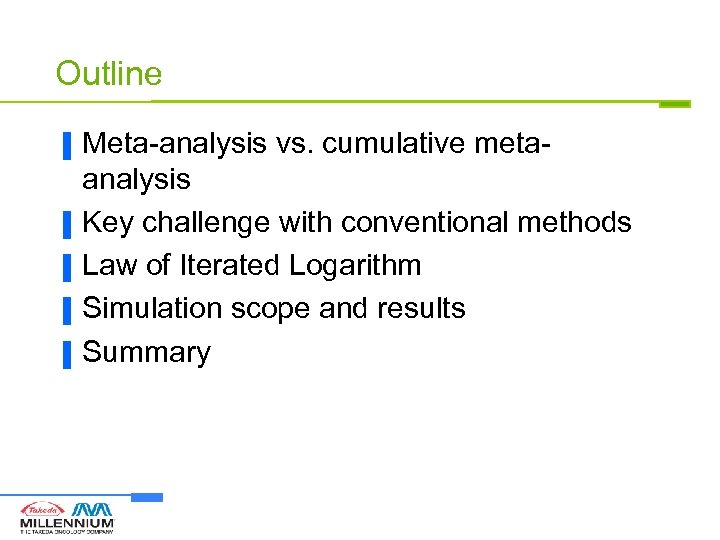Outline ▐ ▐ ▐ Meta-analysis vs. cumulative metaanalysis Key challenge with conventional methods Law of Iterated Logarithm Simulation scope and results Summary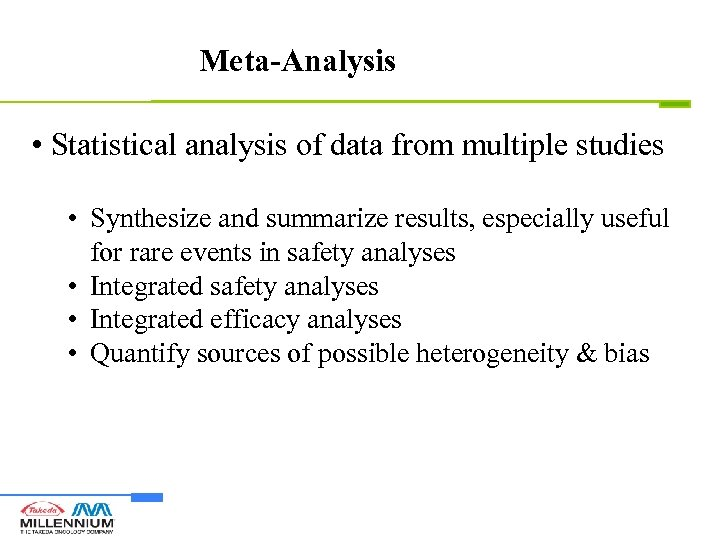Meta-Analysis • Statistical analysis of data from multiple studies • Synthesize and summarize results, especially useful for rare events in safety analyses • Integrated efficacy analyses • Quantify sources of possible heterogeneity & bias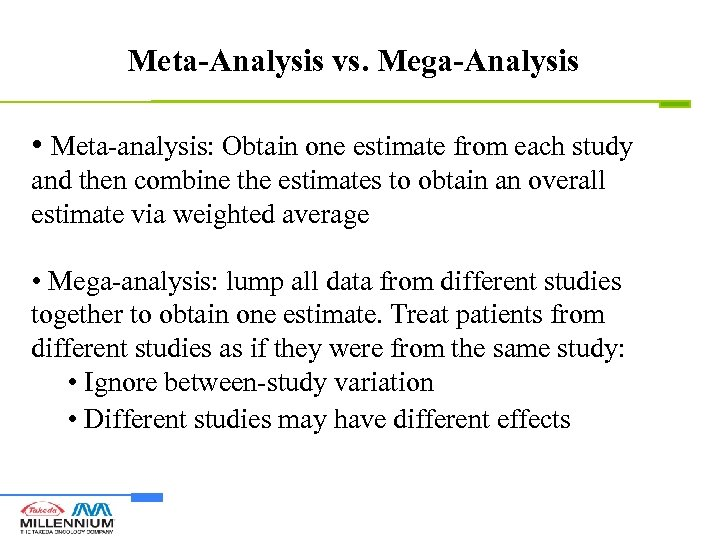Meta-Analysis vs. Mega-Analysis • Meta-analysis: Obtain one estimate from each study and then combine the estimates to obtain an overall estimate via weighted average • Mega-analysis: lump all data from different studies together to obtain one estimate. Treat patients from different studies as if they were from the same study: • Ignore between-study variation • Different studies may have different effects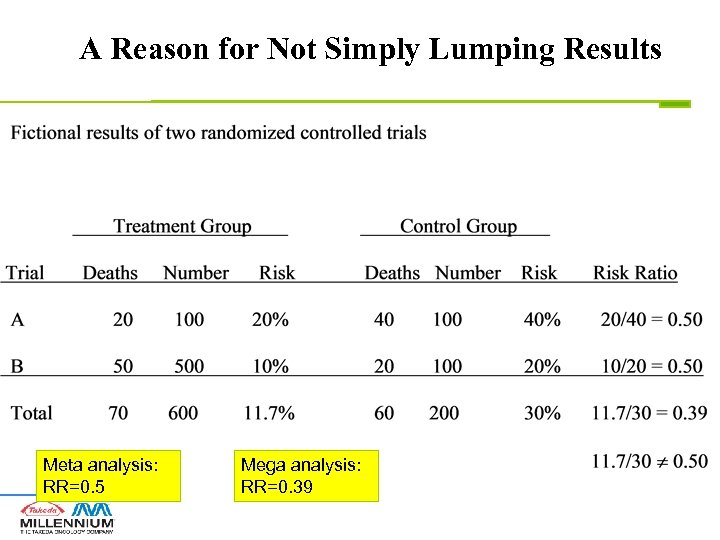A Reason for Not Simply Lumping Results Meta analysis: RR=0. 5 Mega analysis: RR=0. 39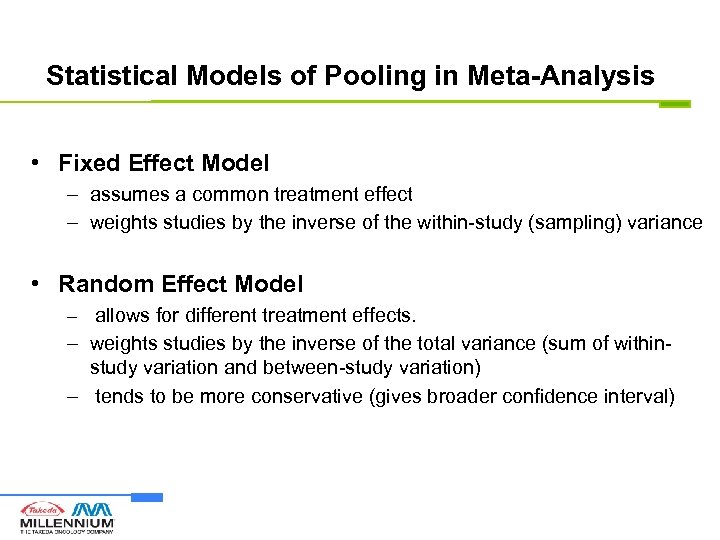Statistical Models of Pooling in Meta-Analysis • Fixed Effect Model – assumes a common treatment effect – weights studies by the inverse of the within-study (sampling) variance • Random Effect Model – allows for different treatment effects. – weights studies by the inverse of the total variance (sum of withinstudy variation and between-study variation) – tends to be more conservative (gives broader confidence interval)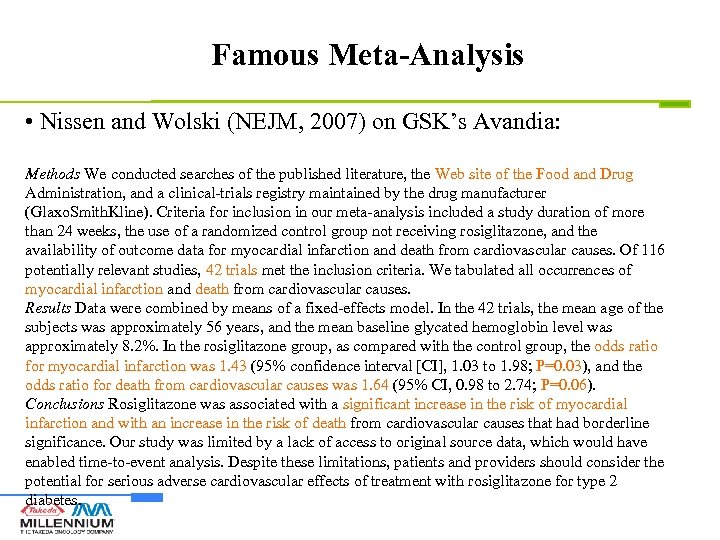Famous Meta-Analysis • Nissen and Wolski (NEJM, 2007) on GSK’s Avandia: Methods We conducted searches of the published literature, the Web site of the Food and Drug Administration, and a clinical-trials registry maintained by the drug manufacturer (Glaxo. Smith. Kline). Criteria for inclusion in our meta-analysis included a study duration of more than 24 weeks, the use of a randomized control group not receiving rosiglitazone, and the availability of outcome data for myocardial infarction and death from cardiovascular causes. Of 116 potentially relevant studies, 42 trials met the inclusion criteria. We tabulated all occurrences of myocardial infarction and death from cardiovascular causes. Results Data were combined by means of a fixed-effects model. In the 42 trials, the mean age of the subjects was approximately 56 years, and the mean baseline glycated hemoglobin level was approximately 8. 2%. In the rosiglitazone group, as compared with the control group, the odds ratio for myocardial infarction was 1. 43 (95% confidence interval [CI], 1. 03 to 1. 98; P=0. 03), and the odds ratio for death from cardiovascular causes was 1. 64 (95% CI, 0. 98 to 2. 74; P=0. 06). Conclusions Rosiglitazone was associated with a significant increase in the risk of myocardial infarction and with an increase in the risk of death from cardiovascular causes that had borderline significance. Our study was limited by a lack of access to original source data, which would have enabled time-to-event analysis. Despite these limitations, patients and providers should consider the potential for serious adverse cardiovascular effects of treatment with rosiglitazone for type 2 diabetes.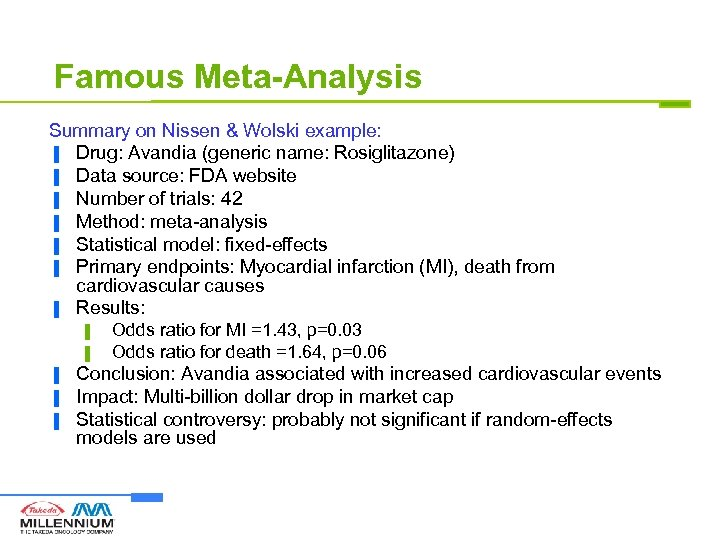Famous Meta-Analysis Summary on Nissen & Wolski example: ▐ Drug: Avandia (generic name: Rosiglitazone) ▐ Data source: FDA website ▐ Number of trials: 42 ▐ Method: meta-analysis ▐ Statistical model: fixed-effects ▐ Primary endpoints: Myocardial infarction (MI), death from cardiovascular causes ▐ Results: ▌ ▌ ▐ ▐ ▐ Odds ratio for MI =1. 43, p=0. 03 Odds ratio for death =1. 64, p=0. 06 Conclusion: Avandia associated with increased cardiovascular events Impact: Multi-billion dollar drop in market cap Statistical controversy: probably not significant if random-effects models are used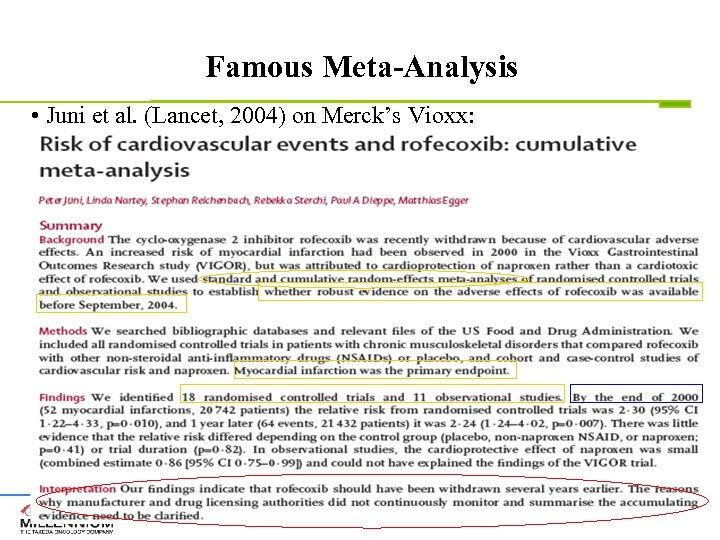Famous Meta-Analysis • Juni et al. (Lancet, 2004) on Merck’s Vioxx: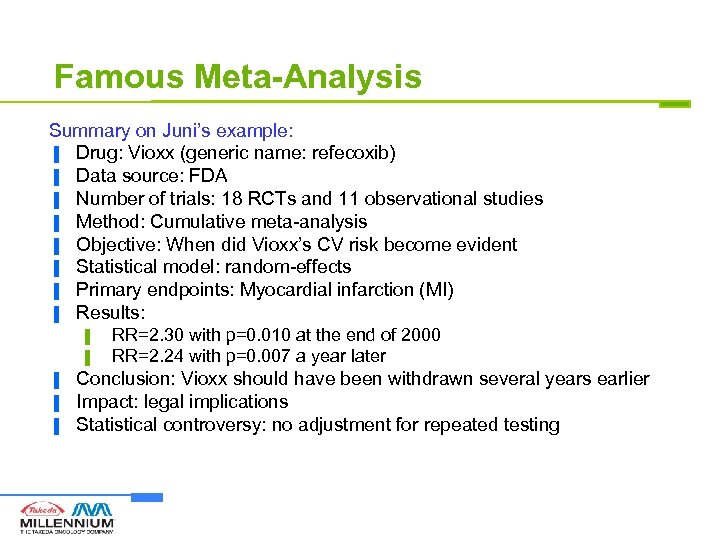Famous Meta-Analysis Summary on Juni’s example: ▐ Drug: Vioxx (generic name: refecoxib) ▐ Data source: FDA ▐ Number of trials: 18 RCTs and 11 observational studies ▐ Method: Cumulative meta-analysis ▐ Objective: When did Vioxx’s CV risk become evident ▐ Statistical model: random-effects ▐ Primary endpoints: Myocardial infarction (MI) ▐ Results: ▌ ▌ ▐ ▐ ▐ RR=2. 30 with p=0. 010 at the end of 2000 RR=2. 24 with p=0. 007 a year later Conclusion: Vioxx should have been withdrawn several years earlier Impact: legal implications Statistical controversy: no adjustment for repeated testing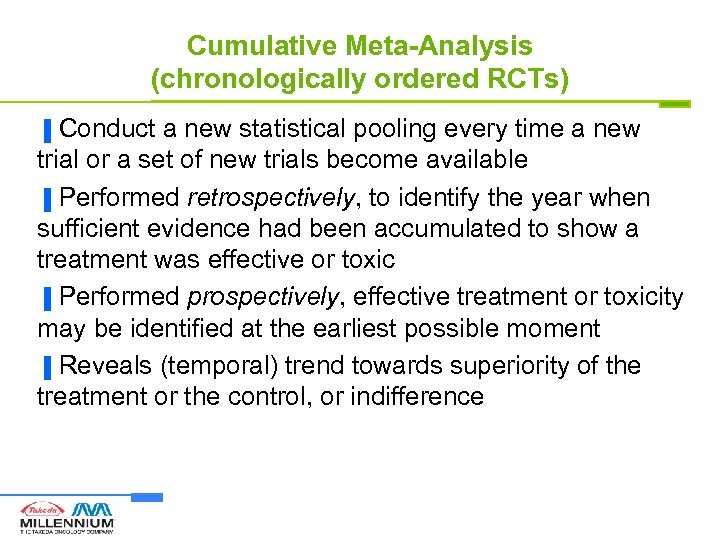Cumulative Meta-Analysis (chronologically ordered RCTs) Conduct a new statistical pooling every time a new trial or a set of new trials become available ▐ Performed retrospectively, to identify the year when sufficient evidence had been accumulated to show a treatment was effective or toxic ▐ Performed prospectively, effective treatment or toxicity may be identified at the earliest possible moment ▐ Reveals (temporal) trend towards superiority of the treatment or the control, or indifference ▐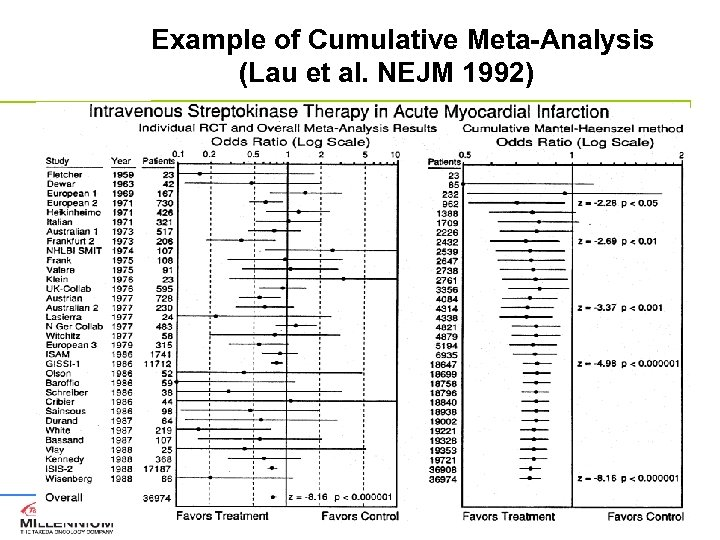Example of Cumulative Meta-Analysis (Lau et al. NEJM 1992)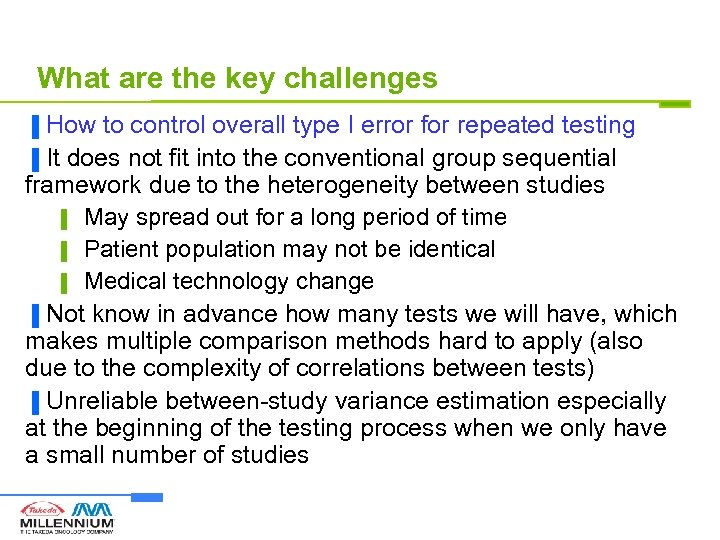What are the key challenges How to control overall type I error for repeated testing ▐ It does not fit into the conventional group sequential framework due to the heterogeneity between studies ▌ May spread out for a long period of time ▌ Patient population may not be identical ▌ Medical technology change ▐ Not know in advance how many tests we will have, which makes multiple comparison methods hard to apply (also due to the complexity of correlations between tests) ▐ Unreliable between-study variance estimation especially at the beginning of the testing process when we only have a small number of studies ▐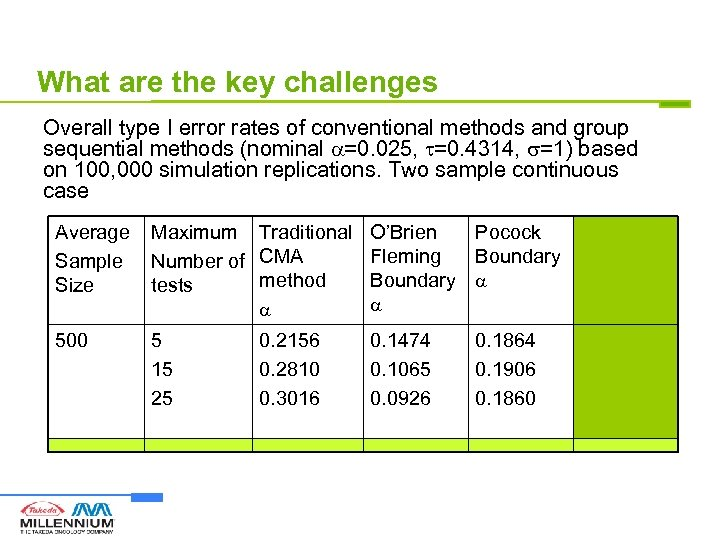What are the key challenges Overall type I error rates of conventional methods and group sequential methods (nominal =0. 025, =0. 4314, =1) based on 100, 000 simulation replications. Two sample continuous case Average Sample Size Maximum Traditional O’Brien Pocock LIL Fleming Boundary Method Number of CMA method Boundary tests 500 5 15 25 0. 2156 0. 2810 0. 3016 0. 1474 0. 1065 0. 0926 0. 1864 0. 1906 0. 1860 0. 0171 0. 0235 0. 0248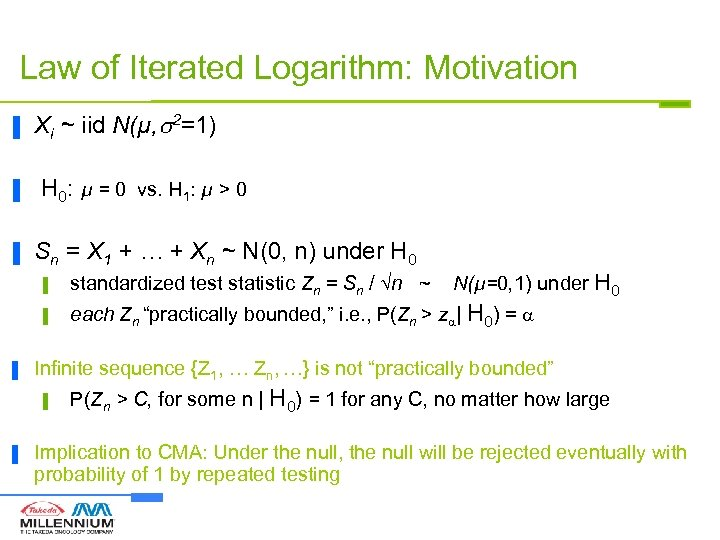Law of Iterated Logarithm: Motivation ▐ ▐ ▐ Xi ~ iid N(µ, 2=1) H 0: µ = 0 vs. H 1: µ > 0 Sn = X 1 + … + Xn ~ N(0, n) under H 0 N(µ=0, 1) under H 0 ▌ ▌ ▐ standardized test statistic Zn = Sn / n ~ each Zn “practically bounded, ” i. e. , P(Zn > z | H 0) = Infinite sequence {Z 1, … Zn, …} is not “practically bounded” ▌ ▐ P(Zn > C, for some n | H 0) = 1 for any C, no matter how large Implication to CMA: Under the null, the null will be rejected eventually with probability of 1 by repeated testing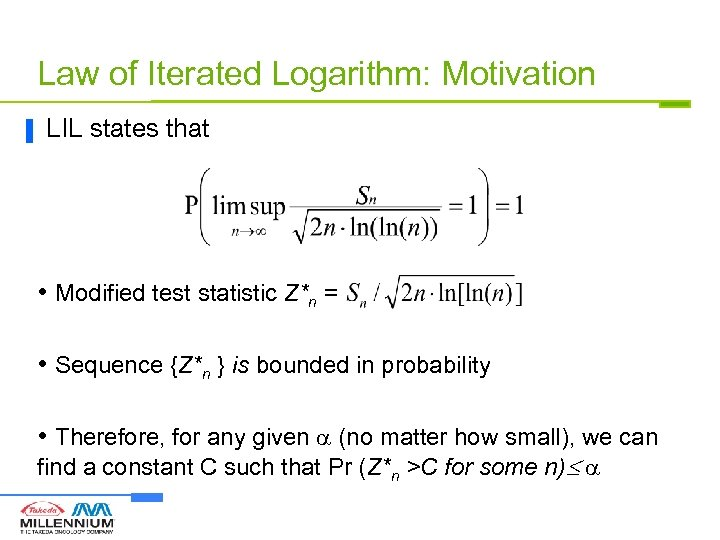Law of Iterated Logarithm: Motivation ▐ LIL states that • Modified test statistic Z*n = • Sequence {Z*n } is bounded in probability • Therefore, for any given (no matter how small), we can find a constant C such that Pr (Z*n >C for some n)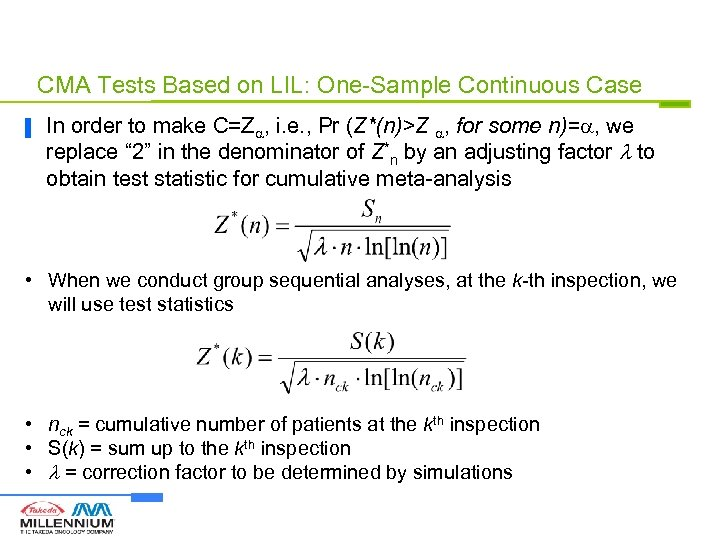CMA Tests Based on LIL: One-Sample Continuous Case ▐ In order to make C=Z , i. e. , Pr (Z*(n)>Z , for some n)= , we replace “ 2” in the denominator of Z*n by an adjusting factor to obtain test statistic for cumulative meta-analysis • When we conduct group sequential analyses, at the k-th inspection, we will use test statistics • nck = cumulative number of patients at the kth inspection • S(k) = sum up to the kth inspection • = correction factor to be determined by simulations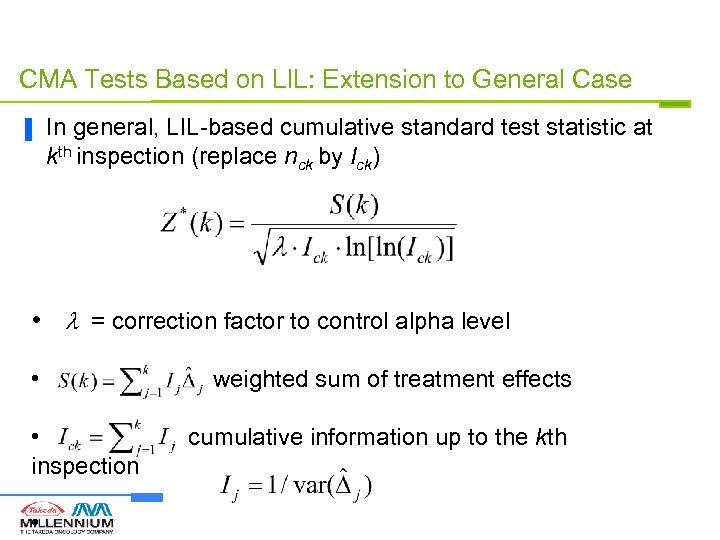CMA Tests Based on LIL: Extension to General Case ▐ In general, LIL-based cumulative standard test statistic at kth inspection (replace nck by Ick) • = correction factor to control alpha level • • inspection • weighted sum of treatment effects cumulative information up to the kth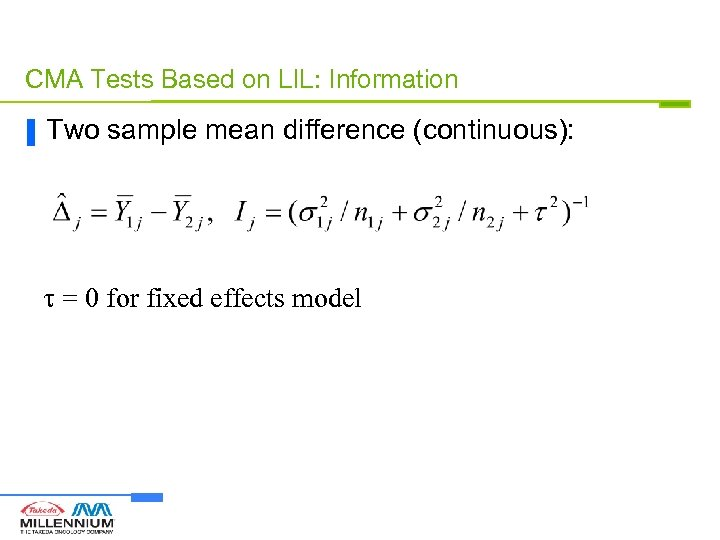CMA Tests Based on LIL: Information ▐ Two sample mean difference (continuous): = 0 for fixed effects model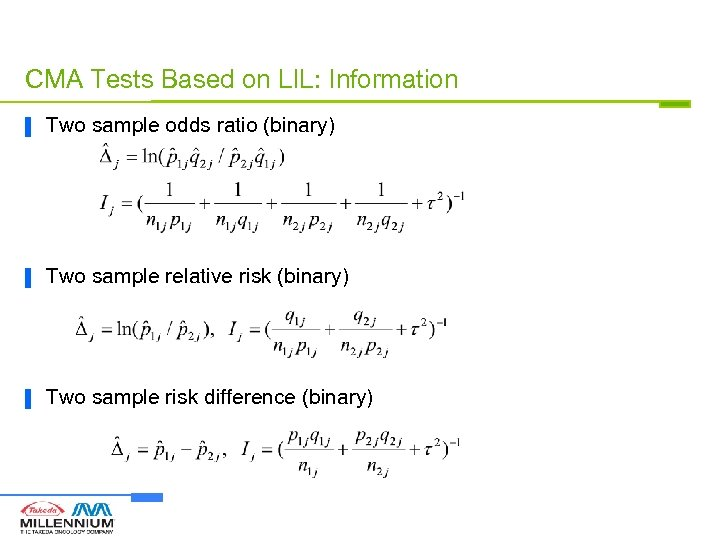CMA Tests Based on LIL: Information ▐ Two sample odds ratio (binary) ▐ Two sample relative risk (binary) ▐ Two sample risk difference (binary)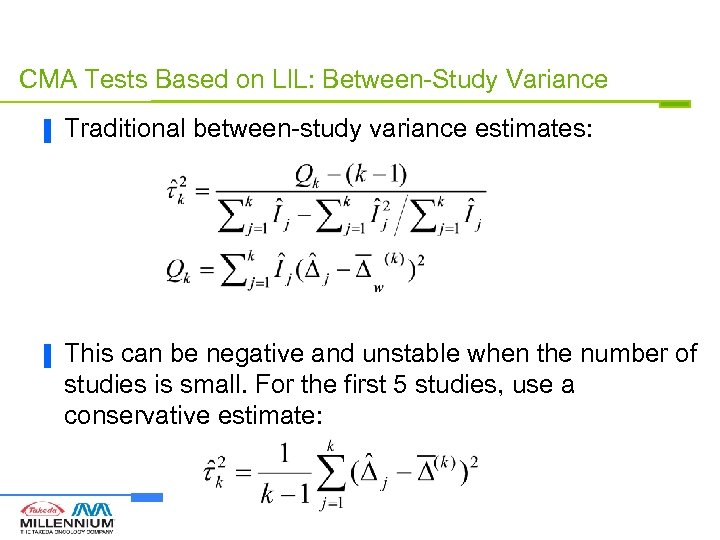CMA Tests Based on LIL: Between-Study Variance ▐ Traditional between-study variance estimates: ▐ This can be negative and unstable when the number of studies is small. For the first 5 studies, use a conservative estimate: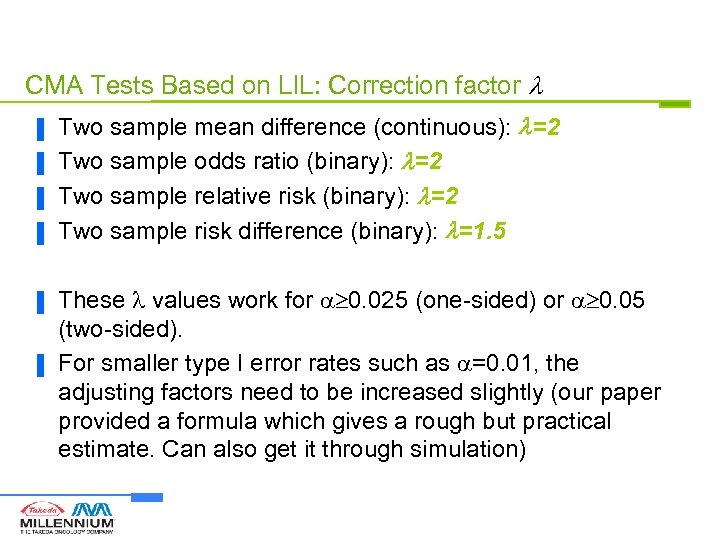CMA Tests Based on LIL: Correction factor ▐ ▐ ▐ Two sample mean difference (continuous): =2 Two sample odds ratio (binary): =2 Two sample relative risk (binary): =2 Two sample risk difference (binary): =1. 5 These values work for 0. 025 (one-sided) or 0. 05 (two-sided). For smaller type I error rates such as =0. 01, the adjusting factors need to be increased slightly (our paper provided a formula which gives a rough but practical estimate. Can also get it through simulation)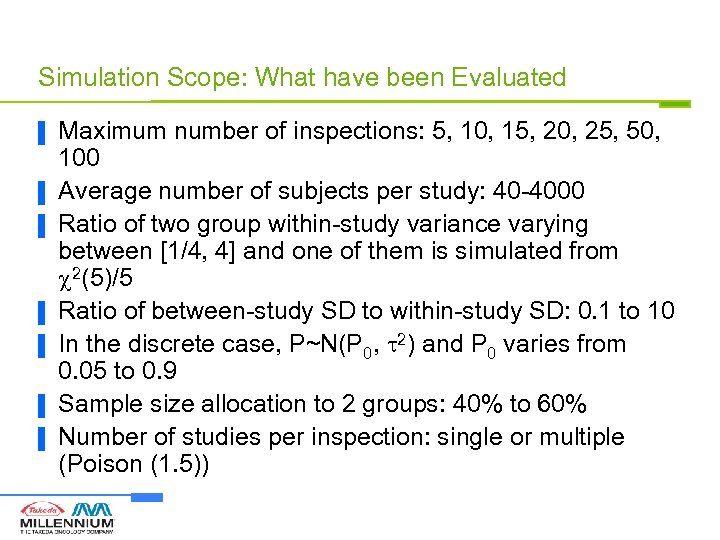Simulation Scope: What have been Evaluated ▐ ▐ ▐ ▐ Maximum number of inspections: 5, 10, 15, 20, 25, 50, 100 Average number of subjects per study: 40 -4000 Ratio of two group within-study variance varying between [1/4, 4] and one of them is simulated from 2(5)/5 Ratio of between-study SD to within-study SD: 0. 1 to 10 In the discrete case, P~N(P 0, 2) and P 0 varies from 0. 05 to 0. 9 Sample size allocation to 2 groups: 40% to 60% Number of studies per inspection: single or multiple (Poison (1. 5))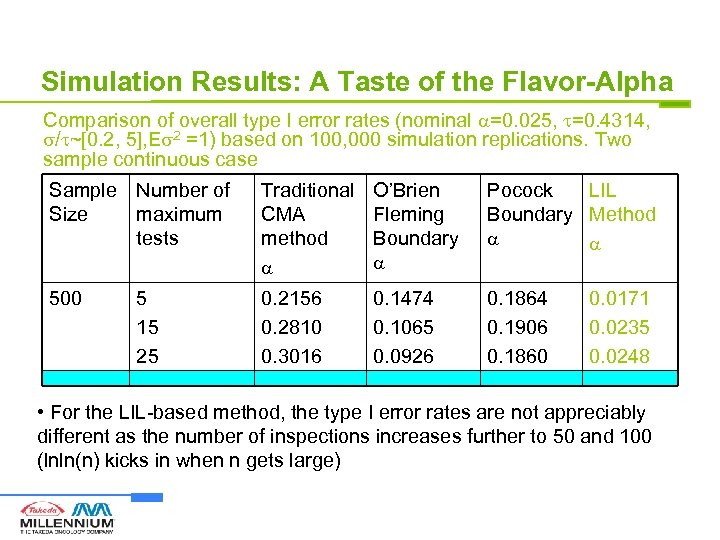Simulation Results: A Taste of the Flavor-Alpha Comparison of overall type I error rates (nominal =0. 025, =0. 4314, / ~[0. 2, 5], E 2 =1) based on 100, 000 simulation replications. Two sample continuous case Sample Number of Size maximum tests Traditional CMA method O’Brien Fleming Boundary Pocock LIL Boundary Method 500 0. 2156 0. 2810 0. 3016 0. 1474 0. 1065 0. 0926 0. 1864 0. 1906 0. 1860 5 15 25 0. 0171 0. 0235 0. 0248 • For the LIL-based method, the type I error rates are not appreciably different as the number of inspections increases further to 50 and 100 (lnln(n) kicks in when n gets large)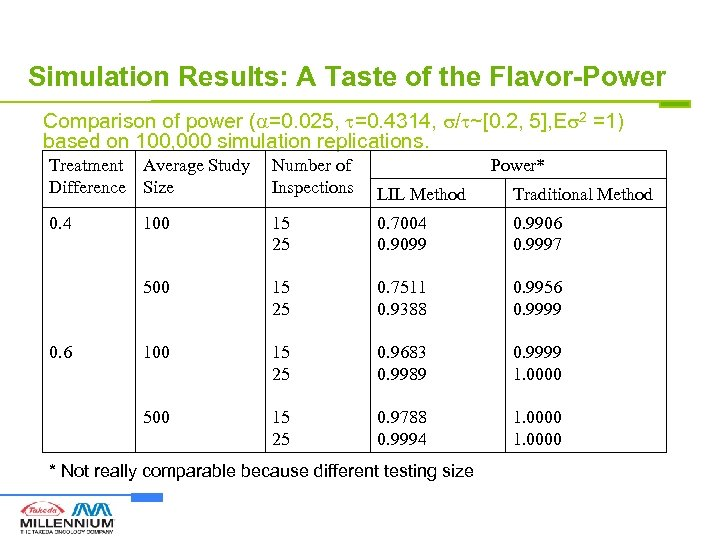Simulation Results: A Taste of the Flavor-Power Comparison of power ( =0. 025, =0. 4314, / ~[0. 2, 5], E 2 =1) based on 100, 000 simulation replications. Treatment Average Study Difference Size Number of Inspections LIL Method Traditional Method 0. 4 100 15 25 0. 7004 0. 9099 0. 9906 0. 9997 500 15 25 0. 7511 0. 9388 0. 9956 0. 9999 100 15 25 0. 9683 0. 9989 0. 9999 1. 0000 500 15 25 0. 9788 0. 9994 1. 0000 0. 6 Power* * Not really comparable because different testing size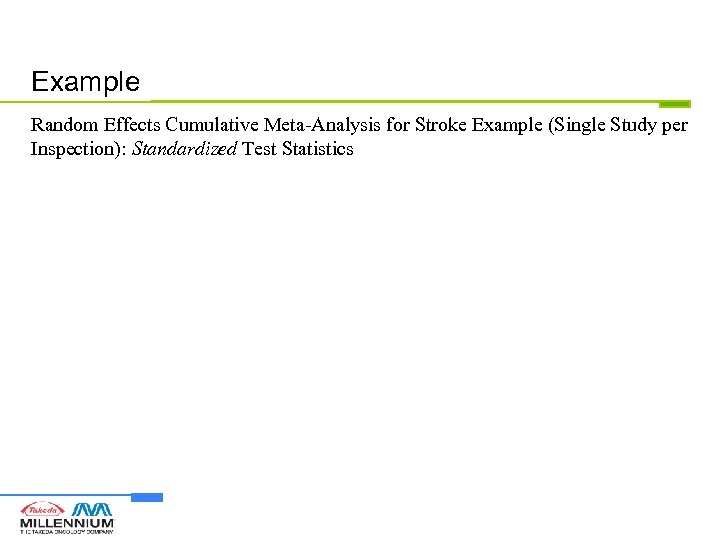Example Random Effects Cumulative Meta-Analysis for Stroke Example (Single Study per Inspection): Standardized Test Statistics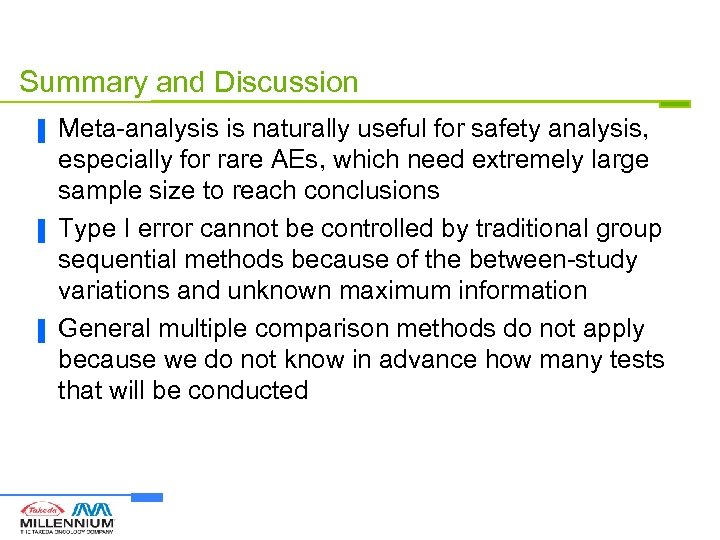Summary and Discussion ▐ ▐ ▐ Meta-analysis is naturally useful for safety analysis, especially for rare AEs, which need extremely large sample size to reach conclusions Type I error cannot be controlled by traditional group sequential methods because of the between-study variations and unknown maximum information General multiple comparison methods do not apply because we do not know in advance how many tests that will be conducted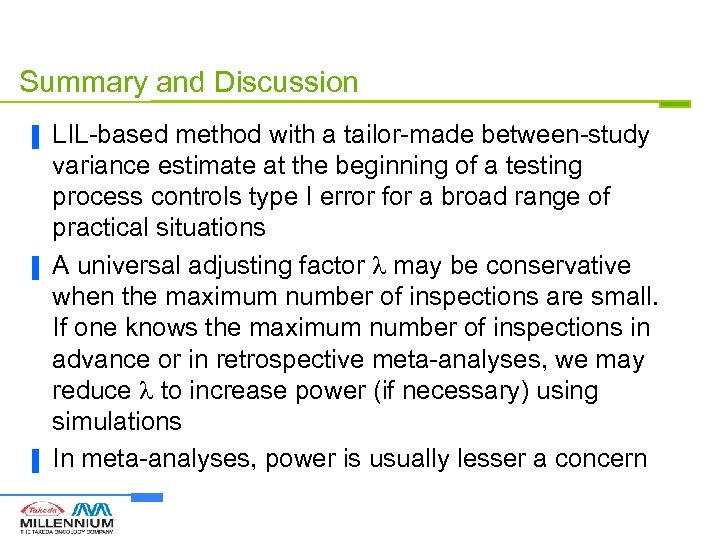Summary and Discussion ▐ ▐ ▐ LIL-based method with a tailor-made between-study variance estimate at the beginning of a testing process controls type I error for a broad range of practical situations A universal adjusting factor may be conservative when the maximum number of inspections are small. If one knows the maximum number of inspections in advance or in retrospective meta-analyses, we may reduce to increase power (if necessary) using simulations In meta-analyses, power is usually lesser a concern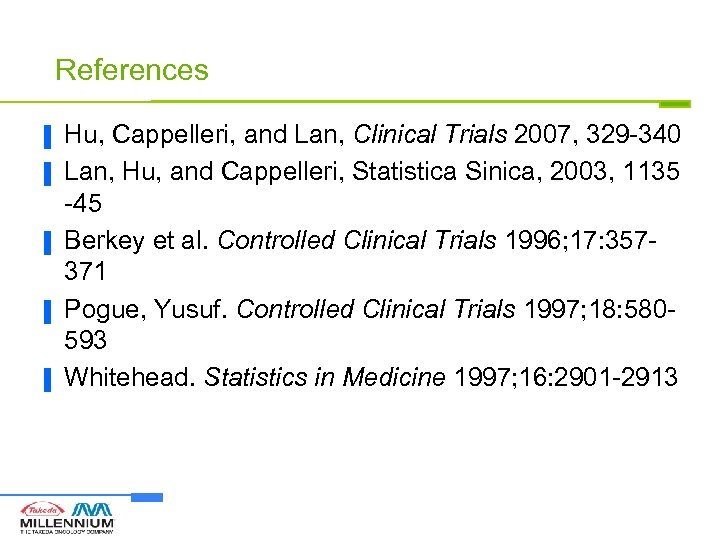References ▐ ▐ ▐ Hu, Cappelleri, and Lan, Clinical Trials 2007, 329 -340 Lan, Hu, and Cappelleri, Statistica Sinica, 2003, 1135 -45 Berkey et al. Controlled Clinical Trials 1996; 17: 357371 Pogue, Yusuf. Controlled Clinical Trials 1997; 18: 580593 Whitehead. Statistics in Medicine 1997; 16: 2901 -2913